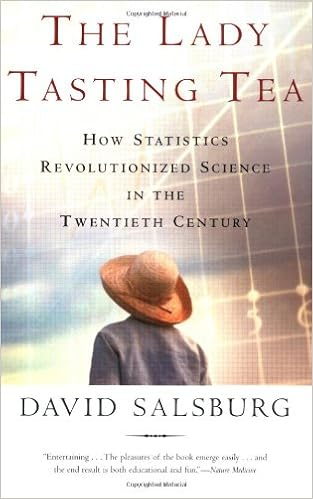R. A. Fisher 的名著《实验设计；第八版，1971年》第二部分有十六页，仅仅讲了一个最简单的实验：女士品茶。这个故事非常有名，以至于 Salsburg 的统计学通俗读物就以它命名：《女士品茶：20世纪统计怎样变革了科学》。Bristol “先奶后茶” Bristol “先茶后奶” 总数
Fisher “先奶后茶” $k$ $4-k$ $4$
Fisher “先茶后奶” $4-k$ $k$ $4$

$$\frac{1}{8 \choose{4}} = \frac{1}{70} = 0.014$$

$$\frac{{4\choose 3} {4 \choose 1}}{8 \choose 4} = \frac{16}{70} = 0.229$$

1. 实验只有一个样本，且不是随机抽取的。
2. 即使我们认为 Bristol 品尝 8 次是 8 个样本，这些样本都是相关的。
3. 更严重的是，前面几杯可能会影响后面几杯的口感，也许会有滞后作用。

y = c(1, 1, 1, 1, 0, 0, 0, 0)
Zpossible = t(combn(8, 4, tabulate, nbins = 8))